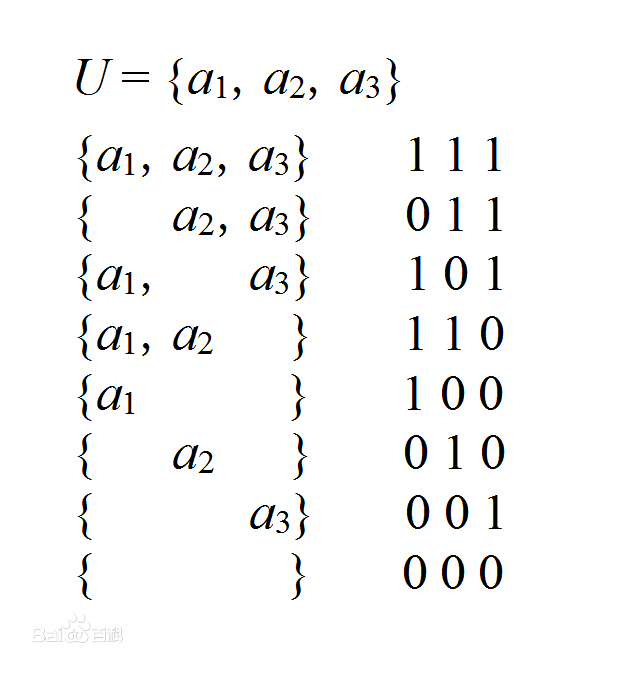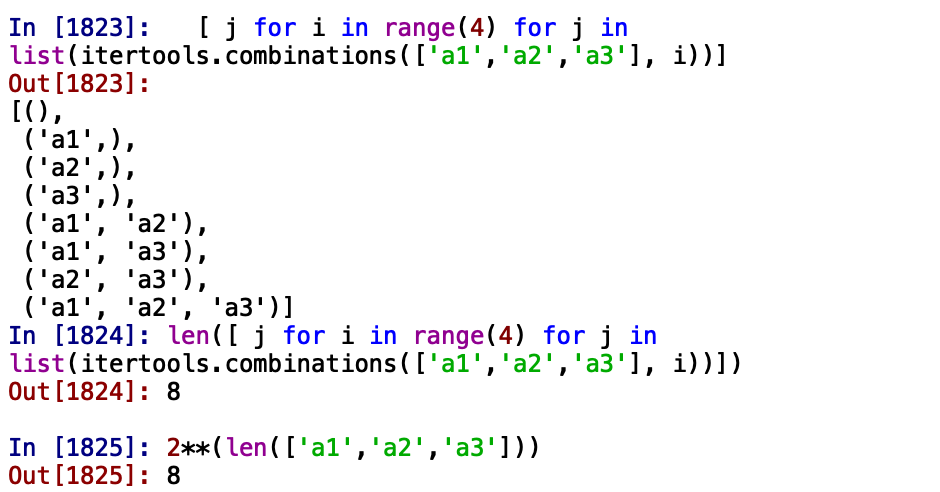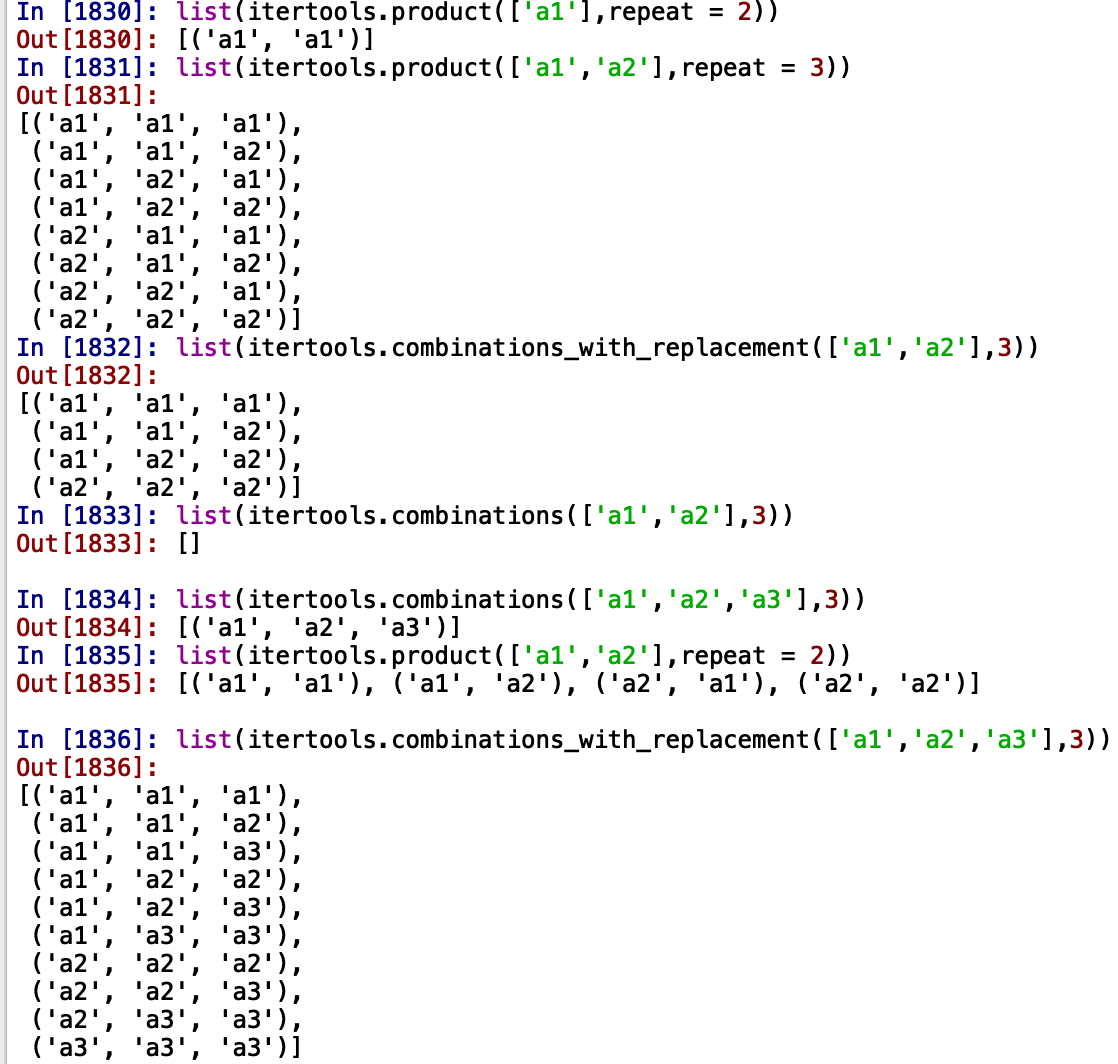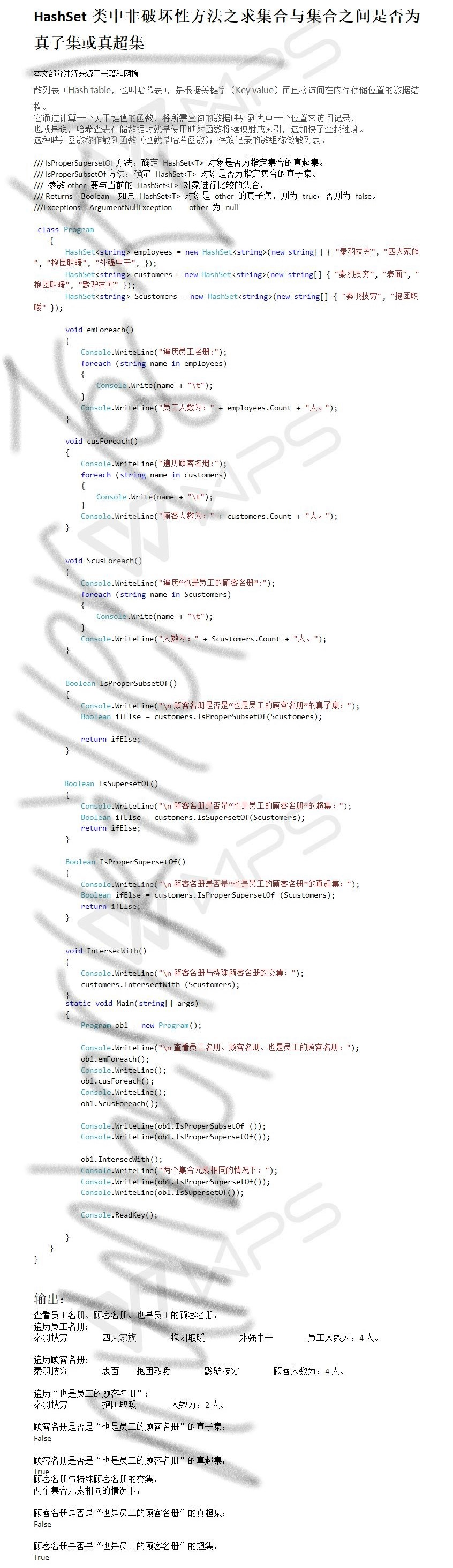• 集合An个元素，则集合A的子集个数为2n，且2n-1个真子集，2n-2个非空真子集。 用python中的itertools.combinations(iterable, r)实现了一下： r: 某特定长度的子序列 另玩了下combinations_with_replacement...

实例:
若集合A有n个元素，则集合A的子集个数为2n，且有2n-1个真子集，2n-2个非空真子集。用python中的itertools.combinations(iterable, r)实现了一下：
r: 某特定长度的子序列另玩了下combinations_with_replacement和 product：展开全文python itertools
• 举例证明可计算数集合是程序数集合真子集，陈必红，，作者发表过一篇论文定义了程序数集合，并证明它是人类社会所能够接触到的实数集合，因此可以提出一个悖论，非程序数不存在。此观
• 本文部分注释来源于书籍网摘 散列表（Hash table，也叫哈希表），是根据关键字（Key value）而直接访问在内存存储位置的数据结构。 它通过计算一个关于键值的函数，将所需查询的数据映射到表中一个位置来访问...
本文部分注释来源于书籍和网摘

散列表（Hash table，也叫哈希表），是根据关键字（Key value）而直接访问在内存存储位置的数据结构。

它通过计算一个关于键值的函数，将所需查询的数据映射到表中一个位置来访问记录，

也就是说，哈希查表存储数据时就是使用映射函数将键映射成索引，这加快了查找速度。

这种映射函数称作散列函数（也就是哈希函数）；存放记录的数组称做散列表。

/// IsProperSupersetOf方法：确定 HashSet<T> 对象是否为指定集合的真超集。

/// IsProperSubsetOf方法：确定 HashSet<T> 对象是否为指定集合的真子集。

/// 参数other 要与当前的 HashSet<T> 对象进行比较的集合。

/// Returns  Boolean  如果 HashSet<T> 对象是 other 的真子集，则为 true；否则为 false。

///Exceptions  ArgumentNullException    other 为 null展开全文C# HashSet
• 一、 真子集 、 二、 空集 、 三、 全集 、 四、 幂集 、 五、 集合元素个数 、 六、 求幂集步骤 、
文章目录一、 真子集二、 空集三、 全集四、 幂集五、 集合元素个数六、 求幂集步骤

一、 真子集

真子集 :
描述 : $A , B$ 两个集合 , 如果 $A$ 集合 是 $B$ 集合的子集 , 并且 $A \not= B$ , 则称 $A$ 是 $B$ 的真子集 , $B$ 真包含 $A$ ;
记作 : $A \subset B$
符号化表示 : $A \subset B$  $\Leftrightarrow$ $A \subseteq B \land A \not= B$

非真子集 :
描述 : $A$ 集合 不是 $B$ 集合的真子集 ;
记作 :  $A \not\subset B$
符号化表示 : $A \not\subset B$  $\Leftrightarrow$ $\exist x ( x \in A \land x \not\in B ) \land A \not= B$
( 存在元素 $x$ 是集合 $A$ 的元素 , 不是集合 $B$ 的元素 , 并且 $A , B$ 不相等 , 则 $A$ 不是 $B$ 的真子集 )

真包含关系 性质 :
反自反性 : $A \not\subset A$
反对称性 : 如果 $A \subset B$ , 那么 $B \not\subset A$
传递性 : 如果 $A \subset B$ , 并且 $B \subset C$ , 那么 $A \subset C$

二、 空集

空集描述 : 没有任何元素的集合 , 称为空集合 , 简称为 空集 ;
记作 : $\varnothing$

空集示例 : $A = \{ x | x^2 + 1 = 0 \land x \in R \}$
$R$ 是实数集合 , 上述 $x$ 明显无解 , 集合也为空集 ;

空集定理 : 空集是一切集合的子集 ;
空集推论 : 空集是唯一的 ;

三、 全集

全集 : 限定所讨论的集合 , 都是某个集合的子集 , 则称该集合为全集 , 记作 $E$ ;

全集不唯一 : 全集只是相对于讨论问题的范畴 , 不唯一 , 不能讨论范畴之外的情况 ;

全集示例 : 讨论 [0, 1] 区间上的实数性质 , 取全集为 [0, 1] 上的所有实数 ;
( 讨论其它区间的数 , 也可以取其它的区间作为全集 )

四、 幂集

幂集描述 : $A$ 是一个集合 , $A$ 集合的全体子集组成的集合 称为 $A$ 的幂集 ;
记作 : $P(A)$
符号化表述 : $P(A) = \{ x | x \subseteq A \}$

五、 集合元素个数

集合元素个数 :
$0$ 元集 : $\varnothing$
$1$ 元集 : 含有 $1$ 个元素的集合 , 又称为 单元集 ;
$2$ 元集 : 含有 $2$ 个元素的集合 ;
$\vdots$
$n$ 元集 : 含有 $n$ 个元素的集合 ; ( $n \geq 1$ )

有穷集 : $|A|$ 表示集合 $A$ 中的元素个数 , 如果 $A$ 集合中的元素个数是 有限数 时 , 那么称该 $A$ 集合为有穷集 , 或 有限集 ;

幂集个数定理 : 集合 $A$ 中的 元素个数 $|A| = n$ , 则 $A$ 的 幂集个数 $|P(A)| = 2^n$ ;

六、 求幂集步骤

求幂集步骤 : 求 集合 $A$ 的幂集 , 需要按照顺序求 $A$ 集合中 由低到高元的所有子集 , 再将这些子集组成集合 ;
低到高元的所有子集 : $0$ 元集 , $1$ 元集 , $2$ 元集 , $\cdots$ , $n$ 元集 ;

集合 $A = \{ a, b , c \}$
$0$ 元集 : $\varnothing$
$1$ 元集 : $\{ a \}$ , $\{ b \}$ , $\{ c \}$
$2$ 元集 : $\{ a, b \}$ , $\{ a, c \}$ , $\{ b, c \}$
$3$ 元集 : $\{ a, b, c \}$
集合 $A$ 的幂集是 :
$P(A) = \{ \varnothing , \{ a \} , \{ b \} , \{ c \} , \{ a, b \} , \{ a, c \} , \{ b, c \} , \{ a, b, c \} \}$


展开全文• 面试中一道笔试题，大概意思如下： ...真子集为不包含子集的集合；非空真子集即不包含子集与空集合 解题思路：这道题可以使用“按位对应法”进行计算如集合A={a,b,c},对于任意一个元素，在每个子集中，要么...
面试中有一道笔试题，大概意思如下：
输入一个集合，输出这个集合的所有子集。例如输入：1，2，4　　输出结果如下所示：
[1, 2][1, 4][2, 4][1, 2, 4]
需要认识的：空集是任何集合的子集；真子集为不包含子集的集合；非空真子集即不包含子集与空集合
解题思路：这道题可以使用“按位对应法”进行计算如集合A={a,b,c},对于任意一个元素，在每个子集中，要么存在，要么不存在。 映射为子集： (a,b,c) (1,1,1)->(a,b,c) (1,1,0)->(a,b) (1,0,1)->(a,c) (1,0,0)->(a) (0,1,1)->(b,c) (0,1,0)->(b) (0,0,1)->(c) (0,0,0)->@(@表示空集) 观察以上规律，与计算机中数据存储方式相似，故可以通过一个整型数与集合映射...000 ~ 111...111（表示有，表示无，反之亦可），通过该整型数逐次增可遍历获取所有的数，即获取集合的相应子集。
主要考察的是位移运算以及逻辑思维能力，具体代码如下（经过本机真实认证，绝对可靠）：

1 import java.util.ArrayList;
2 import java.util.Scanner;
3 import org.apache.commons.collections.CollectionUtils;
4
5 /**
6  * 输入一个集合，输出这个集合的所有子集
7  * @author liangyongxing
8  * @time 2017-02-06
9  */
10 public class SubListExport {
11     public static void main(String[] args) {
12         ArrayList<Integer> list = new ArrayList<Integer>();
13         System.out.println("请输入一串整数并在输入时用英文逗号隔开：");
14         String inputString = new Scanner(System.in).next().toString();
15         if (inputString != null && !inputString.isEmpty()) {
16             String[] strArray = inputString.split(",");
17             for (String str : strArray) {
19             }
20             ArrayList<ArrayList<Integer>> allsubsets = getSubsets(list);
21             for(ArrayList<Integer> subList : allsubsets) {
22                 System.out.println(subList);
23             }
24         }
25     }
26
27     public static ArrayList<ArrayList<Integer>> getSubsets(ArrayList<Integer> subList) {
28         ArrayList<ArrayList<Integer>> allsubsets = new ArrayList<ArrayList<Integer>>();
29         int max = 1 << subList.size();
30         for(int loop = 0; loop < max; loop++) {
31             int index = 0;
32             int temp = loop;
33             ArrayList<Integer> currentCharList = new ArrayList<Integer>();
34             while(temp > 0) {
35                 if((temp & 1) > 0) {
37                 }
38                 temp>>=1;
39                 index++;
45         return allsubsets;
46     }
47 }

注：2017-02-08 10:01:32  上述代码有一定的漏洞即当输入有重复数字的时候，结果会有重复子集输出，并不能满足题目要求，需要在算出子集的时候加入HashSet进行排重，最终打印结果从sets中获取即可，具体修改详情如下图所示：
1. 在主函数打印的地方修改接受的返回值为HashSet类型2. 在函数部分需要修改封装列表有list改为set至此修改完成，测试运行结果如下所示：分析代码可以得出它的时间复杂度是：O(n*log2n)
代码下载地址:https://github.com/liang68/interview 或参看我的个人博客 http://www.cnblogs.com/liang1101/p/6372115.html
@转载请注明
转载于:https://www.cnblogs.com/liang1101/p/6372115.html
展开全文• ## 【子集与真子集】

千次阅读 2019-03-02 20:16:18
子集是包括本身的元素的集合真子集是出自本身的元素的集合。 子集 ：集合A范围大于或等于集合B，B是A的子集； 真子集集合A范围比B大，B是A的真子集例： 举例来说明吧 如集合A={1,2} 则A的子集：空集，{1}，{...
• 若一个集合N个元素 项目 个数 备注 子集 2N2^N2N 包含空本身 真子集 2N−12^N-12N−1 除去本身 非空子集 2N−12^N-12N−1 除去空集 非空真子集 2N−22^N-22N−2 除去本身空集 例子： 集合{a...
• 一、题目：假设递增有序的带头结点的链表A、B分别表示一个集合，试设计算法以判断集合A是否是集合B的子集，如是返回1，否则返回0。二、思路：1.A的值大于B的值，那就A的元素不变，B指向下一个元素，再比较；2.A的值...算法 链表 java 数据结构
• 一个集合，求其全部子集(包含集合自身)。例如集合[1,2,3]其全部子集为：<∅,1,2,12,3,13,23,123> 分析： 方法一：位图法 ①使用两层循环，外层循环为子集个数，对于集合长度为N，子集个数为。外层...c# 算法
• A={1,2,3} B={1,2} print("A与B元素不重复：",A.isdisjoint(B)) print("A是否为B的子集：",A.issubset(B)) print("A是否B的父集：",A.issuperset(B))python
• var subsets = from m in Enumerable.Range(0, 1  select  (from i in Enumerable.Range(0, lstTemp.Count)  where (m &
• 初等数学中的初数知识：数列N={0,1，2...，n，...}没末项；然而可数集概念让N的末项一下子浮出水面推翻百年集论。由中学数学应有的集合起码常识证明无穷集A任一真子集不可～A。
• 之前拿到求组合的题目想法差不多，要用递归来解决。递归的话，思路是这样的：当我遇到一个元素时，我可以考虑把它加入子集，也可以考虑不把它加入子集，到达递归边界时，输出子集的内容。 代码： #include&...
• 求一个集合的所有子集就是很简单的一道关于组合的题目，是一...首先如果一个n个元素的的集合子集的个数一共多少个呢，是2^n个，为什么是2^n个呢，转换一下思路，我们就把n个元素的集合看作是一个n位的整型数...排列组合
• 求一个集合的所有子集 Python实现 #!/usr/bin/env python3 # -*- coding: utf-8 -*- """ Created on Sat Jun 23 16:59:07 2018 @author: luogan """ def PowerSetsBinary...
• 学过回溯法分支限界法之后，觉得对递归了很好地理解，但是让写集合（字符串）的子集和全排列的code时，一时又感觉手足无措。 全排列 基本的想法：将字符串或集合的第一个元素固定，然后让其...递归 数据结构算法
•algorithm recursive c++
• 定义 集合叫做集合子集，记为，当且仅当，即当且仅当
• 我们这些不能出去实习的还是老实的呆在实验室码代码（我们组的同学是好同学，学习认真，工作认真）。 去年参加B公司的一个设计比赛，进了复赛；今天组内组了一个小团队，认真了一次反而落选了，有些遗憾，
• ## 子集和问题

万次阅读 2018-02-07 11:35:34
子集和问题 描述 Description 【问题描述】 　子集和问题的一个实例为〈S,t〉。...　对于给定的正整数的集合S={ x1， x2，…， xn}正整数c，编程计算S 的一个子集S1，使得子集S1等于c。 【输...
• 集合广泛应用于计数问题，这类问题需要讨论集合的大小。 令S为集合。若S中恰n个不同的元素，n是非负整数，就说S是有限集合，而n是S...为此构造一个新的集合，它以S的所有子集作为它的元素，该集合称为S的幂集合，...
• ## 子集

2019-09-05 19:51:12
给定一组不含重复元素的整数数组nums，返回该数组所有可能的子集（幂集）。 说明：解集不能包含重复的子集。 说明：解集不能包含重复的子集。原题 示例： 输入: nums = [1,2,3] 输出: [ , , , [1,2,...
• 感谢刘大大； ...01111仅仅是代表这个集合最大的子集罢了。 然后就是subset函数了，这里面的操作是把二进制转化为十进制的数字。（的，算法的好奇妙啊！）
• 子集 一般地，对于两个集合A、B，如果集合...如果集合A⊆B，存在元素x∈B，且元素x不属于集合A，我们称集合A与集合B真包含关系，集合A是集合B的真子集（proper subset）。记作A⊊B（或B⊋A），读作“A真包含于B”（或包含
• 　今天偶然看到个关于数学中集合的问题，就突发奇想的想用python实现下求一个集合子集。 准备 　我当然先要复习下，什么是集合，什么是子集？ 　比较粗犷的讲法，集合就是一堆确定的东西，细致一点的讲法呢，...
• 问题描述：编写c++递归函数，输出n个元素的所有子集，例如三元素集{a,b,c}的子集是、{a}、{b}、{c}、 {a,b}，{a,c}，{b,c}，{a,b,c}。用0/1组成的代码序来表示分别是000,100,010,001,110,101,011,111（0表示 元素...
• 就以集合中的真子集符号为例，我们怎样才能方便快捷地用MathType数学公式编辑器输入真子集符号。 用MathType输入真子集的方法两种：   方法一：利用工具栏输入  1.打开MathType软件，在上标与下标模板中...MathType 数学公式编辑器...